This is a post in our “equations and shapes” category, where we post articles explaining the relations between equations and the shapes they define.

At school, lines and circles are some of the first geometrical objects you encounter. Later, you also learn that they may be described by equations defining points in a coordinate system. In this post, we review this relationship between lines and circles in 2d and equations briefly with a focus on implicit equations.

In subsequent posts, we will use this to go into 3d space, and explain equations for planes and spheres (i.e., surfaces of balls), and later even for much more involved shapes such as the famous Barth sextic world record surface.

### Straight lines

From school, we know how to interpret equations such as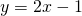in an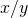-coordinate system. It defines a line with slopemeeting the vertical axis in the point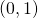.

What you see above is not a static image, but an interactive app! Feel free to play with the parameters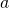andto adapt the equation. Via the white point in the top right grid, you may change bothandat the same time!

Side note: It is beyond the scope of this post to explain how and why the parameters are linked to the line. Just very briefly: chaning the constant term moves the line up and down, the factor in front of theis the slope of the line. If you are interested in more background on this, just send me a message, and I will try to write a post on this in the future.

Have you noticed that it is posssible to define a horizontal line in this way, e.g.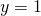(does not appear), but that it is not possible to define a vertical line by a formula of the type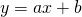in an-coordinate system? This is because the slopehas to be “infinity” () for this special case. But we wish to avoidat this point. One way to do this is to note that a vertical line contains exactly those points which have a common-coordinate. So, we may describe all points on a vertical line with-coordinateby the equation:. In such an equation,does not appear.

In order to be able to treat even such cases without having to think about “infinity”, we may add another parameter in front of the, say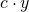, which may be zero or not. The complete equation now reads: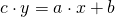. For, we get non-vertical lines as before, and for, we usually get vertical lines.

An even more special case occurs ifand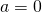. For, this yields the equationwhich is true no matter which values ofandwe choose because these variables do not appear at all, so all points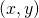in the plane satisfy this equation! And for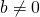, say, we get an equation of the kind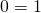which is not true, no matter how we chooseand.

All these cases may be experienced by playing with the parameters in the following app:

### Parabolas

Similarly, for a polynomial of degreein one variable, say, we may consider the graph. It will be a so-called parabola. You may try to understand the effect of the parameters. In some cases, it is not difficult. E.g., changingwill move the graph up and down. Can you describe the effect of parameter? The exact effect of parameteris much more difficult to understand, I think.

Side note: As above, we are not able to go into details about this here because this is standard school material und thus considered as basics for the purpose of this blog. But again, if you are interested in the basics behind this, just send me a message, and I will try to write a post on these basics in the future.

### Circles

In contrast to most of the curves above – but similar to vertical lines – circles may not be defined as a graph of a function of the form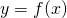for some polynomialin one variablebecause for many valuesthere are two different corresponding values of. But we may still describe any circle by a single polynomial equation thanks to the Pythagorean theorem:

Theorem (usually called “Pythagorean theorem” – to be discussed and proved in a subsequent post):
A triangle with edgesis rectangular with longest edgeif and only if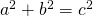.
(In other words, the sum of the areas of the squares over the edgesandis the same as the area of the square over.)

How can we use this now in order to find an equation describing all points on a circle? Let us start with the simplest case where the center of the circle is the origin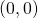of the-coordinate system. Point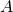in the picture has-coordinateand-coordinate– at least whenis in the upper-right part of the coordinate system; otherwise, one or both coordinates will have an additional minus-sign. But in any case, we may use the Pythagorean theorem to conclude that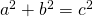.  Now, as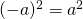and, it follows for any pointon the circle that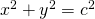, andis the radius of that circle.

In order to generalize this to the case of a circle of radiuswith a center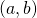not necessarily at the origin, note that for any point, we may consider the translated point. For the centerof the circle, this will be the origin. For any point on the circle around, the translated point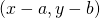will be a point on a circle around the origin, of the same radius. Conversely, any point satisfyinglies on a circle of radiusaround.

### Outlook

In subsequent posts in the “equations and shapes” category, we will use equations for lines, parabolas, and circles as described above for three dimensional objects.

This will go far beyond planes and spheres. One natural class of objects involving the equation of a circle are surfaces of revolution such as hyperboloids, vases, etc.

I hope in the future I will be able to extend my app cwp to allow me to realize some interesting problems you may solve directly within your browser.

Apart from the mathematics, another focus in this category of posts will be the fascinating people and historical developments behind some of the equations and shapes.

### Software used

This post uses our own software cwp to visualize curves with parameters. It is based on the javascript library CindyJS. See Math-Sculpture.com/cwp for details. Other widgets have been created using pure javascript/CindyJS programming, without using our cwp app.